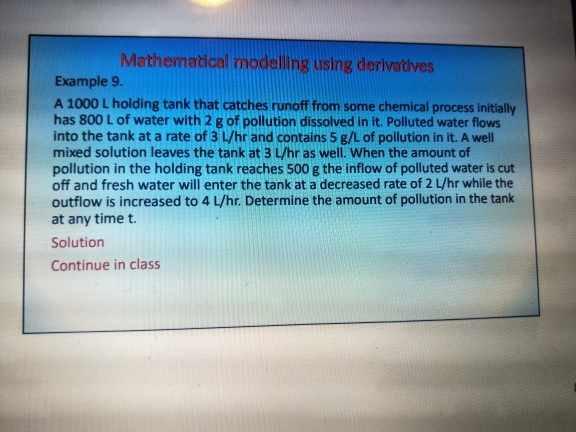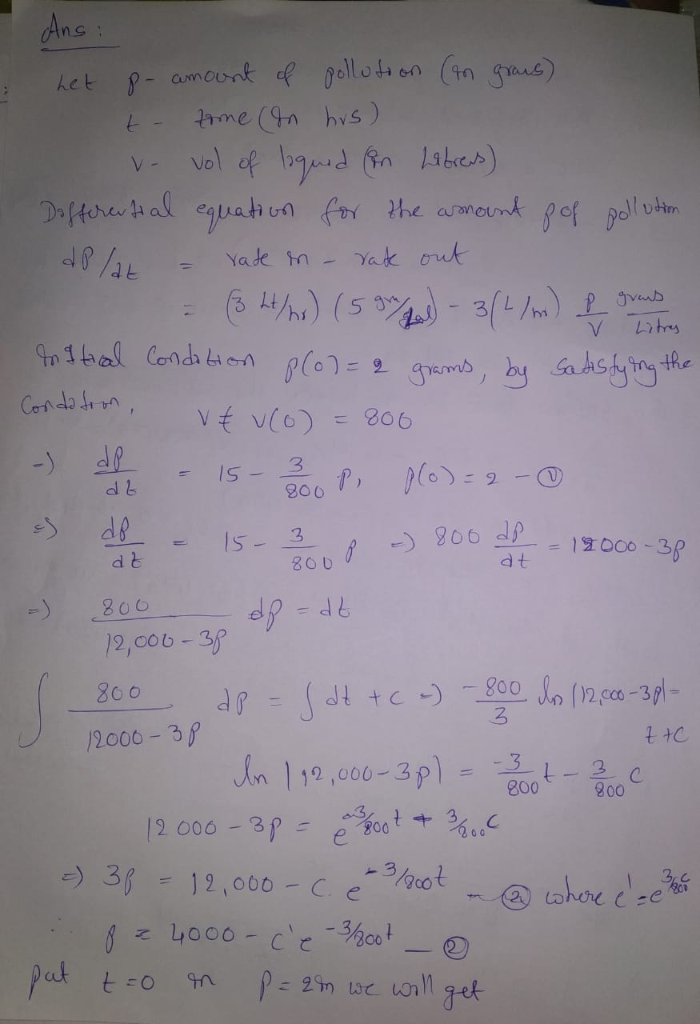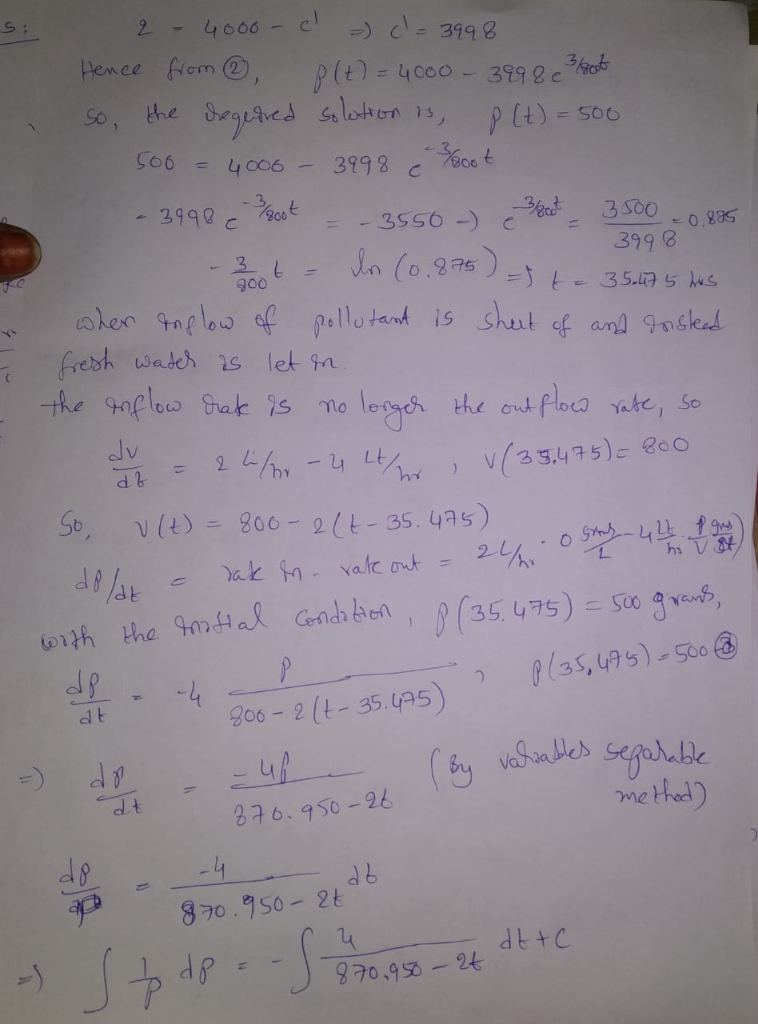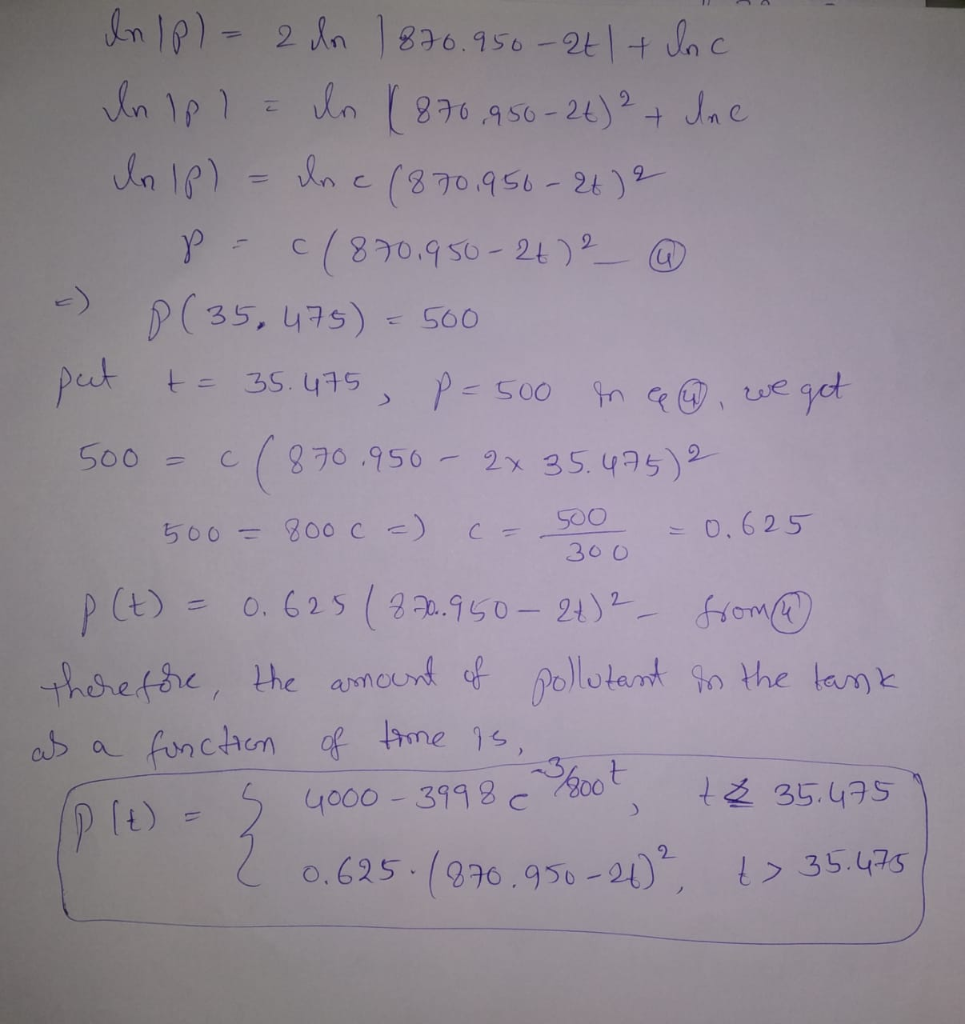# Mathematical modeling using derivatives Example 9 A 1000 L holding tank that catches runoff from ...Mathematical modeling using derivatives Example 9 A 1000 L holding tank that catches runoff from some chemical process initially has 800 L of water with 2 g of pollution dissolved in it. Polluted water flows into the tank at a rate of 3 L/hr and contains 5 g/L of pollution in it. A well mixed solution leaves the tank at 3 L/hr as well. When the amount of pollution in the holding tank reaches 500 g the inflow of polluted water is cut off and fresh water will enter the tank at a decreased rate of 2 L/hr while the outflow is increased to 4 L/hr. Determine the amount of pollution in the tank at any time t. Solution Continue in class##### Add Answer of: Mathematical modeling using derivatives Example 9 A 1000 L holding tank that catches runoff from ...
More Homework Help Questions Additional questions in this topic.